# Down Sample - 2020.2 English

## Vivado Design Suite Reference Guide: Model-Based DSP Design Using System Generator (UG958)

Document ID
UG958
Release Date
2020-11-18
Version
2020.2 English

This block is listed in the following Xilinx® Blockset libraries: Basic Elements and Index.

The Xilinx Down Sample block reduces the sample rate at the point where the block is placed in your design.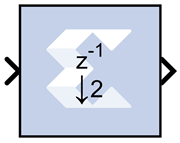The input signal is sampled at even intervals, at either the beginning (first value), or end (last value) of a frame. The sampled value is presented on the output port and held until the next sample is taken.

A Down Sample frame consists of l input samples, where l is sampling rate. An example frame for a Down Sample block configured with a sampling rate of 4 is shown below.

Figure 1. Down Sample Block Example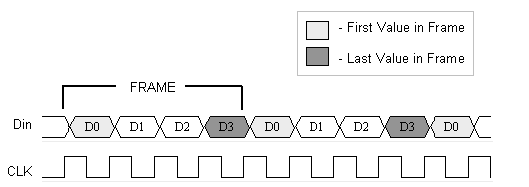The Down Sample block is realized in hardware using one of three possible implementations that vary in terms of implementation efficiency. The block receives two clock enable signals in hardware, Src_CE, and Dest_CE. Src_CE is the faster clock enable signal and corresponds to the input data stream rate. Dest_CE is the slower clock enable, corresponding to the output stream rate, for example, down sampled data. These enable signals control the register sampling in hardware.

## Zero Latency Down Sample

The zero latency Down Sample block must be configured to sample the first value of the frame. The first sample in the input frame passes through the mux to the output port. A register samples this value during the first sample duration and the mux switches to the register output at the start of the second sample of the frame. The result is that the first sample in a frame is present on the output port for the entire frame duration. This is the least efficient hardware implementation as the mux introduces a combinational path from Din to Dout. A single bit register adjusts the timing of the destination clock enable, so that it is asserted at the start of the sample period, instead of the end. The hardware implementation is shown below:

Figure 2. Down Sample with Zero Latency Example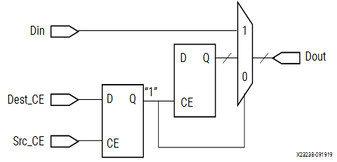## Down Sample with Latency

If the Down Sample block is configured with latency greater than zero, a more efficient implementation is used. One of two implementations is selected depending on whether the Down Sample block is set to sample the first or last value in a frame.

If the block samples the first value in a frame, two registers are required to correctly sample the input stream. The first register is enabled by the adjusted clock enable signal so that it samples the input at the start of the input frame. The second register samples the contents of the first register at the end of the sample period to ensure output data is aligned correctly.

Figure 3. Down Sample with Latency Example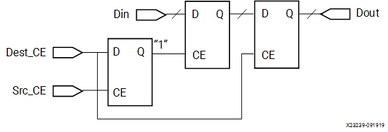If the block samples the last value in a frame, a register samples the data input data at the end of the frame. The sampled value is presented for the duration of the next frame. The most efficient implementation is when the Down Sample block is configured to sample the last value of the frame.

Figure 4. Sampling Last Value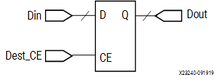## Block Parameters

The block parameters dialog box can be invoked by double-clicking the icon in your Simulink® model.

Basic tab
Sampling Rate (number of input samples per output sample)
Must be an integer greater or equal to 2. This is the ratio of the output sample period to the input, and is essentially a sample rate divider. For example, a ratio of 2 indicates a 2:1 division of the input sample rate. If a non-integer ratio is desired, the Up Sample block can be used in combination with the Down Sample block.
Sample
The Down Sample block can sample either the first or last value of a frame. This parameter will determine which of these two values is sampled.

Other parameters used by this block are explained in the topic Common Options in Block Parameter Dialog Boxes.

## Xilinx LogiCORE

The Down Sample block does not use a Xilinx LogiCORE™.